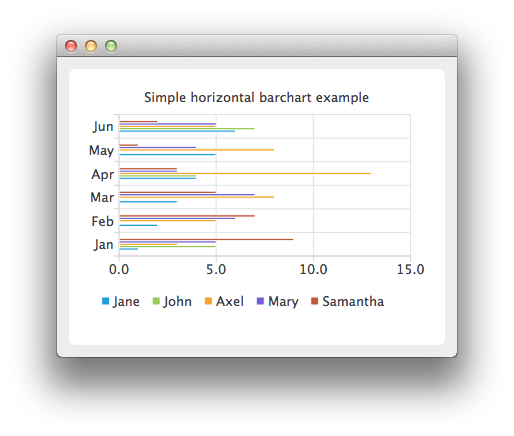# Creating Horizontal Bar Charts

Note: This is part of the Charts with Widgets Gallery example.

QHorizontalBarChart shows the data in sets as separate bars, which are grouped in categories. QHorizontalBarChart works just like QBarChart, except that the bars are drawn horizontally on the chart.The bar sets are used in the same way in all bar charts. To illustrate the difference between various bar charts, we use the same data in all examples. Data that bar chart visualizes, is defined by QBarSet instances. Here we create the sets and append data to them. The data is appended here with << operator. Alternatively, the append method could also be used.

```auto set0 = new QBarSet("Jane");
auto set1 = new QBarSet("John");
auto set2 = new QBarSet("Axel");
auto set3 = new QBarSet("Mary");
auto set4 = new QBarSet("Samantha");

*set0 << 1 << 2 << 3 << 4 << 5 << 6;
*set1 << 5 << 0 << 0 << 4 << 0 << 7;
*set2 << 3 << 5 << 8 << 13 << 8 << 5;
*set3 << 5 << 6 << 7 << 3 << 4 << 5;
*set4 << 9 << 7 << 5 << 3 << 1 << 2;```

We create the series and append the bar sets to it. The series takes ownership of the barsets. The series groups the data from sets to categories. The first values of each set are grouped together in the first category; the second values in the second category, and so on.

```auto series = new QHorizontalBarSeries;
series->append(set0);
series->append(set1);
series->append(set2);
series->append(set3);
series->append(set4);```

Here we create the chart object and add the series to it. We set the title for chart with setTitle and then turn on animations of the series by calling setAnimationOptions(QChart::SeriesAnimations).

```auto chart = new QChart;
chart->setTitle("Simple Horizontal Bar Chart");
chart->setAnimationOptions(QChart::SeriesAnimations);```

To have categories displayed on axis, we need to create a QBarCategoryAxis for that. Here we create a category axis with a list of categories and set it to be aligned to the left-hand side, acting as the y-axis, and attach it to the series. The chart takes ownership of the axis. For x-axis we use a value axis, aligned to the bottom.

```QStringList categories {"Jan", "Feb", "Mar", "Apr", "May", "Jun"};
auto axisY = new QBarCategoryAxis;
axisY->append(categories);
series->attachAxis(axisY);
auto axisX = new QValueAxis;
series->attachAxis(axisX);
axisX->applyNiceNumbers();```

We also want to show the legend. To do so, we get the legend pointer from chart and set it to visible. We also place the legend to the bottom of the chart by setting its alignment to Qt::AlignBottom.

```chart->legend()->setVisible(true);
chart->legend()->setAlignment(Qt::AlignBottom);```

Finally we add the chart onto a view. We also turn on the antialiasing for the chartView.

`createDefaultChartView(chart);`

The chart is ready to be shown.